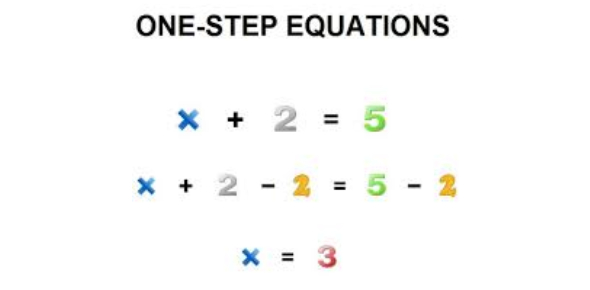# Solving One Step Equations Containing Integers Quiz!

10 Questions | Attempts: 7147SettingsAn integer is a number that has no fractional part and no digits after the decimal point. This quiz has been created for students and learners to learn the basic concepts of solving one-step equations including Multiplication, Division, Addition, and Subtraction. Read the questions carefully and answer. Read the questions carefully and answer. So, let's try out the quiz. All the best!

• 1.
H - 15 = -17
• A.

H = -2

• B.

H = 2

• C.

H = 39

• D.

H = -39

• 2.
A - 29 = -12
• A.

A = 41

• B.

A = -41

• C.

A = 17

• D.

A = -17

• 3.
13 = g - 20
• A.

G = 7

• B.

G = 33

• C.

G = -7

• D.

G = -33

• 4.
M + 48 = 31
• A.

M = 79

• B.

M = 17

• C.

M = -79

• D.

M = -17

• 5.
0 = z + 50
• A.

Z = 50

• B.

Z = 0

• C.

Z = -50

• D.

Z = -100

• 6.
15y = -75
• A.

Y = 5

• B.

Y = -60

• C.

Y = -5

• D.

Y = 60

• 7.
0 = -7d
• A.

D = 0

• B.

D = 1

• C.

D = -1

• D.

D = 7

• 8.
R / 4 = -250
• A.

R = 100

• B.

R= -100

• C.

R = 1000

• D.

R = -1000

• 9.
T / -11 = 11
• A.

T = -1

• B.

T = -121

• C.

T = 11

• D.

T = -11

• 10.
Compare the following Integers. -6 _______-20
• A.

<

• B.

>

• C.

=

• D.

None of the above

## Related TopicsBack to top
×

Wait!
Here's an interesting quiz for you.